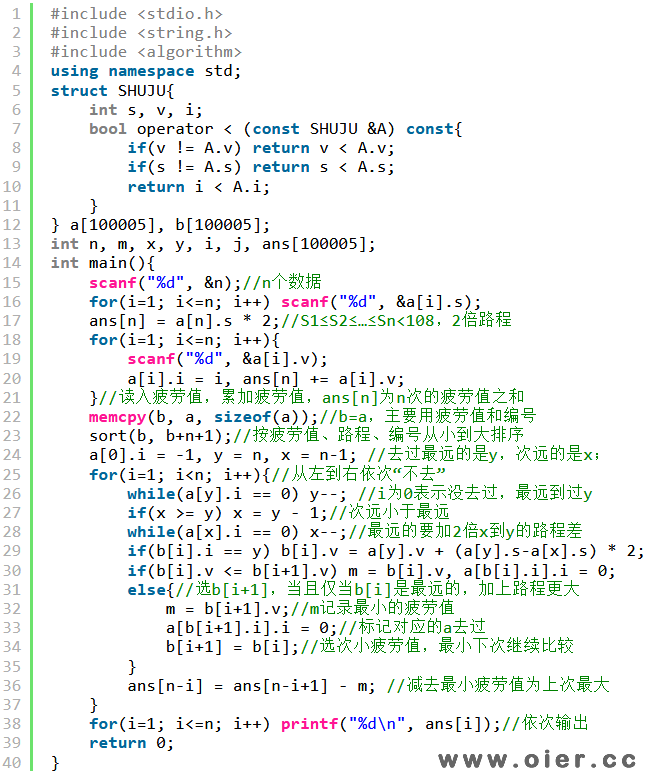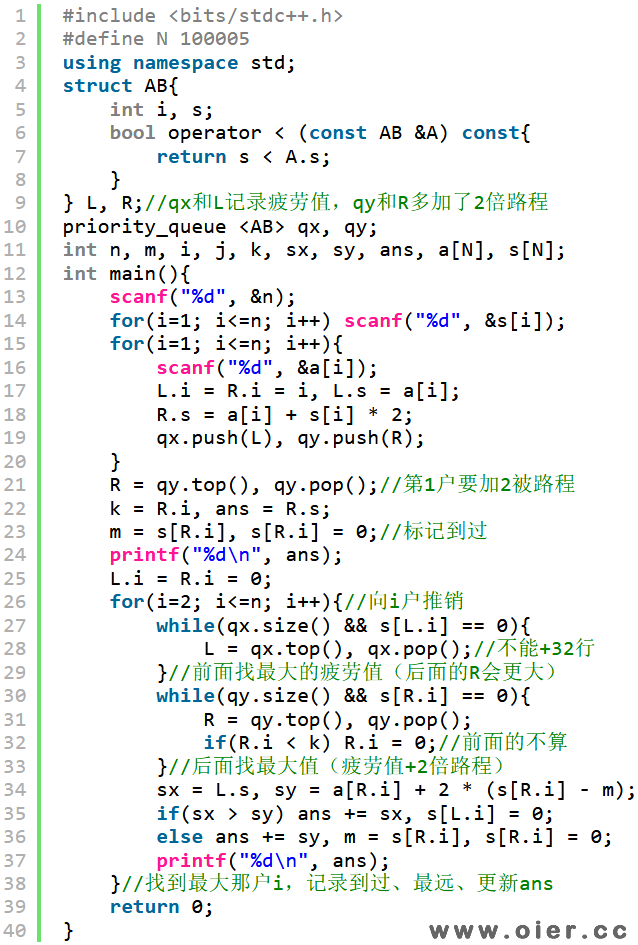SSOJ1411推销员（NOIP2015）
1392+

## 样例输入

5
1 2 3 4 5
1 2 3 4 5

5
1 2 2 4 5
5 4 3 4 1

## 样例输出

15
19
22
24
25

12
17
21
24
27

## 提示

【输入输出样例 1 说明】

X=1: 向住户 5 推销，往返走路的疲劳值为 5+5，推销的疲劳值为 5，总疲劳值为 15。

X=2: 向住户 4、5 推销，往返走路的疲劳值为 5+5，推销的疲劳值为 4+5，总疲劳 值为 5+5+4+5=19。

X=3: 向住户 3、4、5 推销，往返走路的疲劳值为 5+5，推销的疲劳值 3+4+5，总疲 劳值为 5+5+3+4+5=22。

X=4: 向住户 2、3、4、5 推销，往返走路的疲劳值为 5+5，推销的疲劳值 2+3+4+5， 总疲劳值 5+5+2+3+4+5=24。

X=5: 向住户 1、2、3、4、5 推销，往返走路的疲劳值为 5+5，推销的疲劳值 1+2+3+4+5， 总疲劳值 5+5+1+2+3+4+5=25。

【输入输出样例 2 说明】

X=1：向住户 4 推销，往返走路的疲劳值为 4+4，推销的疲劳值为 4，总疲劳值 4+4+4=12。

X=2：向住户 1、4 推销，往返走路的疲劳值为 4+4，推销的疲劳值为 5+4，总疲劳值4+4+5+4=17。

X=3：向住户 1、2、4 推销，往返走路的疲劳值为 4+4，推销的疲劳值为 5+4+4，总疲劳值 4+4+5+4+4=21。

X=4：向住户 1、2、3、4 推销，往返走路的疲劳值为 4+4，推销的疲劳值为 5+4+3+4， 总疲劳值 4+4+5+4+3+4=24。或者向住户 1、2、4、5 推销，往返走路的疲劳值为 5+5，推销的疲劳值为 5+4+4+1，总疲劳值 5+5+5+4+4+1=24。

X=5：向住户 1、2、3、4、5 推销，往返走路的疲劳值为 5+5，推销的疲劳值为 5+4+3+4+1， 总疲劳值 5+5+5+4+3+4+1=27。

NOIP2015普及组第四题

# 程序实现